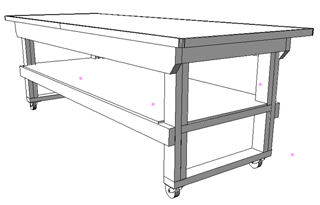# The “Golden Ratio” for Designing Furniture?I would appreciate it if anyone could provide the formula for calculating golden ratio proportions when designing furniture. I have an old article on golden ratio, but it doesn’t describe a ‘how-to,’ step-by-step approach.The problem I encounter is that a piece of furniture’s intended use usually dictates either a height, width or depth requirement. I know one or two of these dimensional requirements, and would appreciate a formula that supplies me with a ‘golden’ aspect when either height or width are known.

Ellis Walentine: The golden ratio is a constant: 1.618. What’s odd about it, among other things, is that .618 is to 1 as 1 is to 1.618. Divide the height of your dresser or table by this number to arrive at the prescribed width.

Michael Dresdner: Fair enough. The ratio of height to width or width to height (either way works) is 1.618 to 1 (or simply .618:1 ? same thing). Simply put, pick your width and multiply it by .618 to get the height, or vice versa. I often cheat by approximating it with any two numbers from the Fibonacci sequence. It works once you get above 8 and is close enough to the golden mean to pass.

For the non-mathematical (read: non-geeks) out there, you generate a Fibonacci sequence by adding the last two numbers in the sequence to get the next number. Starting with 1, it would be as follows: 1, 2, 3, 5, 8, 13, 21, 34, 55, 89, and so on. (3 = 2+1, 5 = 3+2, 8 = 5+3, etc.) Take any two adjacent numbers – 21 and 34 for instance – and you will find they closely approximate the golden mean ratio.